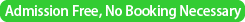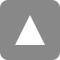﻿ リスク解析戦略研究センター・セミナー / Seminar by Prof. Alan Welsh | 統計数理研究所

# リスク解析戦略研究センター・セミナー / Seminar by Prof. Alan Welsh

Date&Time
2017年3月16日(木) / Mar 16(Thu), 2017
13:30－14:30Place

The Institute of Statistical Mathematics, Tokyo, Japan. Seminar room5 (3F)
Speaker
Alan Welsh (The Australian National University, Canberra, Australia)
Title
The effect of the working correlation on fitting models to longitudinal data
Abstract

Marginal regression models for longitudinal data are specified through the regression function and the co- variance matrix. The most popular method of estimating the regression parameter in these models is the method of Generalized Estimating Equations (GEE, ). The generalized estimating equations estimators are obtained by solving a set of unbiased estimating equations which incorporate a user specified working cor- relation matrix. If the working correlation matrix involves unknown parameters, these are estimated through additional estimating equations. Quadratic Inference Function (QIF) estimators  estimate the regression parameter by minimizing a quadratic function of the terms in an expansion of the generalized estimating equation obtained by expanding the inverse working correlation matrix in terms of basis matrices. The basis matrices do not involve any unknown parameters from the working correlation matrix so these estimators have the advantage of not requiring the estimation of any such unknown parameters, although they may need to be estimated for inference. Quadratic inference function estimators were introduced by  to improve on the efficiency of generalized estimating equations estimators and  argued that quadratic inference func- tion estimators are also robust against outliers, making them preferable to generalized estimating equations estimators.

In this talk, we discuss the properties of quadratic inference function estimators and compare them with generalized estimating equations estimators. In particular, we focus on the effects different working correlation structures have on the computation and the robustness properties of the estimators. The main technical tool is the influence function of the estimators. Our approach is to explore particular cases in detail to gain insights and understanding. We show that the effect of the choice of working correlation is more interesting than is generally believed and we had anticipated.

References
1. Liang, K.Y. & Zeger, S.L. (1986). Longitudinal data analysis using generalized linear models. Biometrika, 78, 13–22.
2. Qu, A., Lindsay, B.G. & Li, B. (2000). Improving generalized estimating equations using quadratic inference functions. Biometrika, 87, 823–836.
3. Qu, A. & Song, P.X.-K. (2004). Assessing robustness of generalized estimating equations and quadratic inference functions. Biometrika, 91, 447–459.